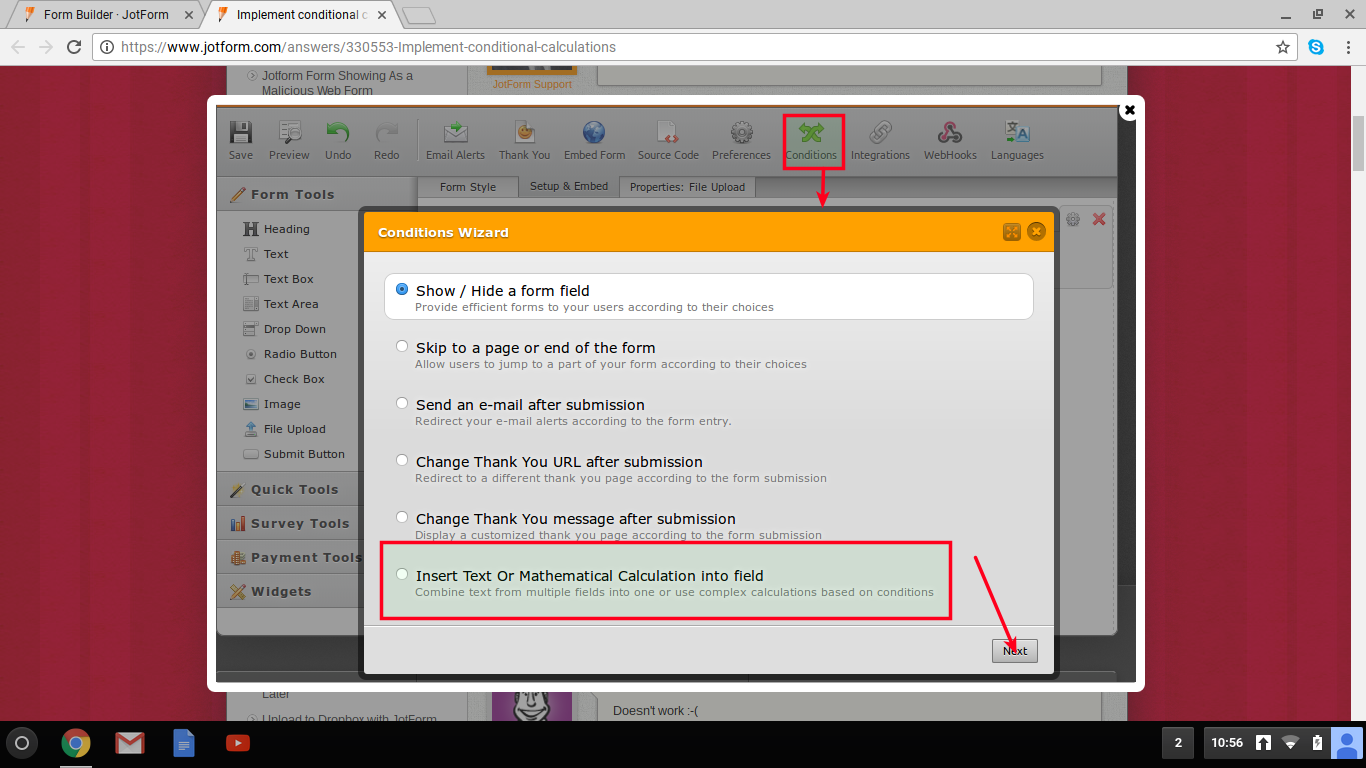Where do I find the inserttext or mathematical calculation

•oklife
Asked on December 17, 2016 at 11:11 PM

i am trying to do a condition whereby if  a certain number of items where selected they will have varying prices. for example if 10 products were selected it will cost \$5 but if 20 products where selected it will cost \$10 instead. thanks

also where can i find that 'insert text or mathematical function into field" check the screenshot•oklife
Answered on December 17, 2016 at 11:17 PM

i am on the starter plan and i do not make use of the product crator i.e i created the form myself.

•EltonCris
Answered on December 18, 2016 at 03:11 AM

Here's how to perform a calculation in your form https://www.jotform.com/help/259-How-to-Perform-Form-Calculation-Using-a-Widget.

With regards to the screenshot, that's updated to a new version. Follow this guide to learn how to perform insert text and mathematical calculation condition https://www.jotform.com/help/268-How-to-Insert-Text-or-Mathematical-Calculation-into-a-Field-Using-Conditional-Logic